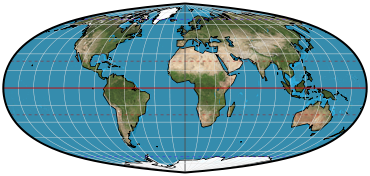## Directory of Map Projections

What is a projection?

Previous | Next

sinucyliParameters: Weight of sinusoidal, Weighted axis, φ₁, Vertical stretch of sinusoidal

Description

Sinucyli is a fusion of the sinusoidal and Lambert cylindric equal-area projections.

Classifications

pseudocylindric
equal-area

Graticule

Meridians: Complex curves equally spaced along parallels.
Parallels: Straight parallel lines.
Poles: Points or lines depending on configuration.
Symmetry: Bilaterally symmetric.

Limiting forms

Sinsoidal, when “Weight of sinusoidal” = 1.0, φ₁ = 0.0, “Weighted axis” = Horizontal, “Vertical stretch” = 1.0.
Lambert cylindric equal-area, when “Weight of sinusoidal” = 0.0, φ₁ = 0.0, “Weighted axis” = Horizontal, “Vertical stretch” = 1.0.
Nell–Hammer, when “Weight of sinusoidal” = 0.5, φ₁ = 0.0, “Weighted axis” = Horizontal, “Vertical stretch” = 1.0.

Scale

Correct along the two parallels of latitude ±φ₁, which is configurable, when “Vertical stretch” = 1.0.

Distortion

No distortion along the two parallels of latitude ±φ₁. As with any pseudocylindric, distortion increases toward outer meridians.

Parameters

Weight of sinusoidal: The fraction of weighting, from [0…1], given to sinusoidal over Lambert equal-area.
Weighted axis: Horizontal or vertical, which axis to perform the averaging between the two projections.
φ₁: The latitudes north and south of the equator at which the projection has no distortion.
Vertical stretch of sinusoidal: How much to stretch the sinusoidal before performing the averaging.

Origin

Developed by Daniel “daan” Strebe (1962–) in 2015.